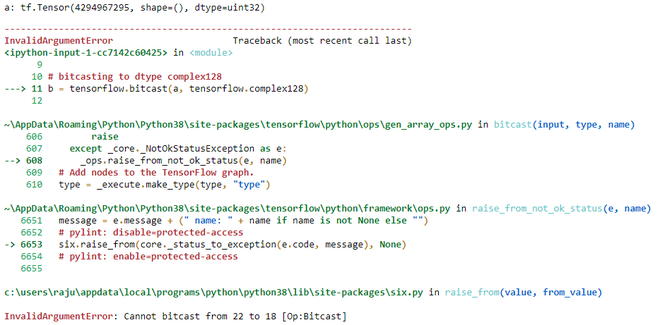Related Articles

# Python | tensorflow.bitcast() method

• Last Updated : 04 Jun, 2020

TensorFlow is open-source python library designed by Google to develop Machine Learning models  and deep learning  neural networks.

bitcast() is method in tensorflow library which is used to bitcast a tensor from one type to another type. It doesn’t copy the data.

```Syntax:
tf.bitcast(
input, type, name
)

Arguments:
1. input: It is the Tensor and the allowed type for this tensor are
bfloat16, half, float32, float64, int64, int32, uint8, uint16, uint32,
uint64, int8, int16, complex64, complex128, qint8, quint8, qint16, quint16, qint32.
2. type: It defines the dtype in which input need to be bitcasted.
3. name: It is an optional argument. It is used to give a name to operation.

Return: It returns a tensor of type type.
```

Note: bitcast can’t be used to cast real dtype to complex dtype. It will raise InvalidArgumentError.

Example 1:

## Python3

 `# importing the library``import` `tensorflow`` ` `# initializing the constant tensor of dtype unit32``a ``=` `tensorflow.constant(``0xffffffff``, dtype``=``tensorflow.uint32)`` ` `# Checking the initialized tensor``print``(``'a:'``,a)`` ` `# bitcasting to dtype unit8``b ``=` `tensorflow.bitcast(a, tensorflow.uint8)`` ` `# Checking the bitcasted tensor``print``(``'b:'``,b)`

Output:

```a: tf.Tensor(4294967295, shape=(), dtype=uint32)
b: tf.Tensor([255 255 255 255], shape=(4,), dtype=uint8)
```

Example 2:

This example tries to bitcast a real dtype to complex dtype

## Python3

 `# importing the library``import` `tensorflow`` ` `# initializing the constant tensor of dtype unit32``a ``=` `tensorflow.constant(``0xffffffff``, dtype``=``tensorflow.uint32)`` ` `# Checking the initialized tensor``print``(``'a:'``,a)`` ` `# bitcasting to dtype complex128``b ``=` `tensorflow.bitcast(a, tensorflow.complex128)`

Output:Attention geek! Strengthen your foundations with the Python Programming Foundation Course and learn the basics.

To begin with, your interview preparations Enhance your Data Structures concepts with the Python DS Course. And to begin with your Machine Learning Journey, join the Machine Learning – Basic Level Course

My Personal Notes arrow_drop_up